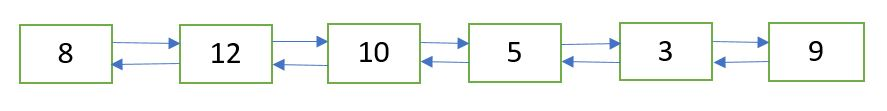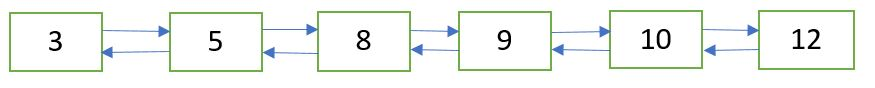Open In App

# Insertion Sort for Doubly Linked List

Sort the doubly linked list using the insertion sort technique.Doubly Linked List after applying insertion sortAlgorithm:
Below is a simple insertion sort algorithm for doubly-linked lists.
1) Create an empty sorted (or result) doubly linked list.
2) Traverse the given doubly linked list, and do the following for every node.
a) Insert the current node in a sorted way in the sorted(or result) doubly linked list.
3) Change the head of the given linked list to the head of the sorted (or result) list.
The main step is (2. a), which has been covered in the post below.
Sorted Insert for Doubly Linked List

## C++

 `// C++ implementation for insertion Sort``// on a doubly linked list``#include ` `using` `namespace` `std;` `// Node of a doubly linked list``struct` `Node {``    ``int` `data;``    ``struct` `Node* prev, *next;``};` `// function to create and return a new node``// of a doubly linked list``struct` `Node* getNode(``int` `data)``{``    ``// allocate node``    ``struct` `Node* newNode =``          ``(``struct` `Node*)``malloc``(``sizeof``(``struct` `Node));` `    ``// put in the data``    ``newNode->data = data;``    ``newNode->prev = newNode->next = NULL;``    ``return` `newNode;``}` `// function to insert a new node in sorted way in``// a sorted doubly linked list``void` `sortedInsert(``struct` `Node** head_ref, ``struct` `Node* newNode)``{``    ``struct` `Node* current;` `    ``// if list is empty``    ``if` `(*head_ref == NULL)``        ``*head_ref = newNode;` `    ``// if the node is to be inserted at the beginning``    ``// of the doubly linked list``    ``else` `if` `((*head_ref)->data >= newNode->data) {``        ``newNode->next = *head_ref;``        ``newNode->next->prev = newNode;``        ``*head_ref = newNode;``    ``}` `    ``else` `{``        ``current = *head_ref;` `        ``// locate the node after which the new node``        ``// is to be inserted``        ``while` `(current->next != NULL &&``               ``current->next->data < newNode->data)``            ``current = current->next;` `        ``/*Make the appropriate links */` `        ``newNode->next = current->next;` `        ``// if the new node is not inserted``        ``// at the end of the list``        ``if` `(current->next != NULL)``            ``newNode->next->prev = newNode;` `        ``current->next = newNode;``        ``newNode->prev = current;``    ``}``}` `// function to sort a doubly linked list using insertion sort``void` `insertionSort(``struct` `Node** head_ref)``{``    ``// Initialize 'sorted' - a sorted doubly linked list``    ``struct` `Node* sorted = NULL;` `    ``// Traverse the given doubly linked list and``    ``// insert every node to 'sorted'``    ``struct` `Node* current = *head_ref;``    ``while` `(current != NULL) {` `        ``// Store next for next iteration``        ``struct` `Node* next = current->next;` `        ``// removing all the links so as to create 'current'``        ``// as a new node for insertion``        ``current->prev = current->next = NULL;` `        ``// insert current in 'sorted' doubly linked list``        ``sortedInsert(&sorted, current);` `        ``// Update current``        ``current = next;``    ``}` `    ``// Update head_ref to point to sorted doubly linked list``    ``*head_ref = sorted;``}` `// function to print the doubly linked list``void` `printList(``struct` `Node* head)``{``    ``while` `(head != NULL) {``        ``cout << head->data << ``" "``;``        ``head = head->next;``    ``}``}` `// function to insert a node at the beginning of``// the doubly linked list``void` `push(``struct` `Node** head_ref, ``int` `new_data)``{``    ``/* allocate node */``    ``struct` `Node* new_node =``         ``(``struct` `Node*)``malloc``(``sizeof``(``struct` `Node));` `    ``/* put in the data  */``    ``new_node->data = new_data;` `    ``/* Make next of new node as head and previous as NULL */``    ``new_node->next = (*head_ref);``    ``new_node->prev = NULL;` `    ``/* change prev of head node to new node */``    ``if` `((*head_ref) != NULL)``        ``(*head_ref)->prev = new_node;` `    ``/* move the head to point to the new node */``    ``(*head_ref) = new_node;``}` `// Driver program to test above``int` `main()``{``    ``/* start with the empty doubly linked list */``    ``struct` `Node* head = NULL;` `    ``// insert the following data``    ``push(&head, 9);``    ``push(&head, 3);``    ``push(&head, 5);``    ``push(&head, 10);``    ``push(&head, 12);``    ``push(&head, 8);` `    ``cout << ``"Doubly Linked List Before Sortingn"``;``    ``printList(head);` `    ``insertionSort(&head);` `    ``cout << ``"nDoubly Linked List After Sortingn"``;``    ``printList(head);` `    ``return` `0;``}`

## Java

 `// Java implementation for insertion Sort``// on a doubly linked list``class` `Solution``{` `// Node of a doubly linked list``static` `class` `Node``{``    ``int` `data;``    ``Node prev, next;``};` `// function to create and return a new node``// of a doubly linked list``static` `Node getNode(``int` `data)``{``    ``// allocate node``    ``Node newNode = ``new` `Node();` `    ``// put in the data``    ``newNode.data = data;``    ``newNode.prev = newNode.next = ``null``;``    ``return` `newNode;``}` `// function to insert a new node in sorted way in``// a sorted doubly linked list``static` `Node sortedInsert(Node head_ref, Node newNode)``{``    ``Node current;` `    ``// if list is empty``    ``if` `(head_ref == ``null``)``        ``head_ref = newNode;` `    ``// if the node is to be inserted at the beginning``    ``// of the doubly linked list``    ``else` `if` `((head_ref).data >= newNode.data)``    ``{``        ``newNode.next = head_ref;``        ``newNode.next.prev = newNode;``        ``head_ref = newNode;``    ``}` `    ``else``    ``{``        ``current = head_ref;` `        ``// locate the node after which the new node``        ``// is to be inserted``        ``while` `(current.next != ``null` `&&``            ``current.next.data < newNode.data)``            ``current = current.next;` `        ``//Make the appropriate links /` `        ``newNode.next = current.next;` `        ``// if the new node is not inserted``        ``// at the end of the list``        ``if` `(current.next != ``null``)``            ``newNode.next.prev = newNode;` `        ``current.next = newNode;``        ``newNode.prev = current;``    ``}``    ``return` `head_ref;``}` `// function to sort a doubly linked list using insertion sort``static` `Node insertionSort(Node head_ref)``{``    ``// Initialize 'sorted' - a sorted doubly linked list``    ``Node sorted = ``null``;` `    ``// Traverse the given doubly linked list and``    ``// insert every node to 'sorted'``    ``Node current = head_ref;``    ``while` `(current != ``null``)``    ``{` `        ``// Store next for next iteration``        ``Node next = current.next;` `        ``// removing all the links so as to create 'current'``        ``// as a new node for insertion``        ``current.prev = current.next = ``null``;` `        ``// insert current in 'sorted' doubly linked list``        ``sorted=sortedInsert(sorted, current);` `        ``// Update current``        ``current = next;``    ``}` `    ``// Update head_ref to point to sorted doubly linked list``    ``head_ref = sorted;``    ` `    ``return` `head_ref;``}` `// function to print the doubly linked list``static` `void` `printList(Node head)``{``    ``while` `(head != ``null``)``    ``{``        ``System.out.print(head.data + ``" "``);``        ``head = head.next;``    ``}``}` `// function to insert a node at the beginning of``// the doubly linked list``static` `Node push(Node head_ref, ``int` `new_data)``{``    ``// allocate node /``    ``Node new_node = ``new` `Node();` `    ``// put in the data /``    ``new_node.data = new_data;` `    ``// Make next of new node as head and previous as null /``    ``new_node.next = (head_ref);``    ``new_node.prev = ``null``;` `    ``// change prev of head node to new node /``    ``if` `((head_ref) != ``null``)``        ``(head_ref).prev = new_node;` `    ``// move the head to point to the new node /``    ``(head_ref) = new_node;``    ` `    ``return` `head_ref;``}` `// Driver code``public` `static` `void` `main(String args[])``{``    ``// start with the empty doubly linked list /``    ``Node head = ``null``;` `    ``// insert the following data``    ``head=push(head, ``9``);``    ``head=push(head, ``3``);``    ``head=push(head, ``5``);``    ``head=push(head, ``10``);``    ``head=push(head, ``12``);``    ``head=push(head, ``8``);` `    ``System.out.println( ``"Doubly Linked List Before Sorting\n"``);``    ``printList(head);` `    ``head=insertionSort(head);` `    ``System.out.println(``"\nDoubly Linked List After Sorting\n"``);``    ``printList(head);` `}``}` `// This code is contributed by Arnab Kundu`

## Python3

 `# Python3 implementation for insertion Sort``# on a doubly linked list` `# Node of a doubly linked list``class` `Node:``    ` `    ``def` `__init__(``self``, data):``        ``self``.data ``=` `data``        ``self``.prev ``=` `None``        ``self``.``next` `=` `None` `# function to create and return a new node``# of a doubly linked list``def` `getNode(data):` `    ``# allocate node``    ``newNode ``=` `Node(``0``)` `    ``# put in the data``    ``newNode.data ``=` `data``    ``newNode.prev ``=` `newNode.``next` `=` `None``    ``return` `newNode` `# function to insert a new node in sorted way in``# a sorted doubly linked list``def` `sortedInsert(head_ref, newNode):` `    ``current ``=` `None` `    ``# if list is empty``    ``if` `(head_ref ``=``=` `None``):``        ``head_ref ``=` `newNode` `    ``# if the node is to be inserted at the beginning``    ``# of the doubly linked list``    ``elif` `((head_ref).data >``=` `newNode.data) :``        ``newNode.``next` `=` `head_ref``        ``newNode.``next``.prev ``=` `newNode``        ``head_ref ``=` `newNode``    ` `    ``else` `:``        ``current ``=` `head_ref` `        ``# locate the node after which the new node``        ``# is to be inserted``        ``while` `(current.``next` `!``=` `None` `and``            ``current.``next``.data < newNode.data):``            ``current ``=` `current.``next` `        ``"""Make the appropriate links """``        ``newNode.``next` `=` `current.``next` `        ``# if the new node is not inserted``        ``# at the end of the list``        ``if` `(current.``next` `!``=` `None``):``            ``newNode.``next``.prev ``=` `newNode` `        ``current.``next` `=` `newNode``        ``newNode.prev ``=` `current``    ` `    ``return` `head_ref;``    ` `# function to sort a doubly linked list``# using insertion sort``def` `insertionSort( head_ref):` `    ``# Initialize 'sorted' - a sorted``    ``# doubly linked list``    ``sorted` `=` `None` `    ``# Traverse the given doubly linked list``    ``# and insert every node to 'sorted'``    ``current ``=` `head_ref``    ``while` `(current !``=` `None``) :` `        ``# Store next for next iteration``        ``next` `=` `current.``next` `        ``# removing all the links so as to create``        ``# 'current' as a new node for insertion``        ``current.prev ``=` `current.``next` `=` `None` `        ``# insert current in 'sorted' doubly linked list``        ``sorted` `=` `sortedInsert(``sorted``, current)` `        ``# Update current``        ``current ``=` `next``    ` `    ``# Update head_ref to point to``    ``# sorted doubly linked list``    ``head_ref ``=` `sorted``    ` `    ``return` `head_ref` `# function to print the doubly linked list``def` `printList(head):` `    ``while` `(head !``=` `None``) :``        ``print``( head.data, end ``=` `" "``)``        ``head ``=` `head.``next``    ` `# function to insert a node at the``# beginning of the doubly linked list``def` `push(head_ref, new_data):` `    ``""" allocate node """``    ``new_node ``=` `Node(``0``)``    ` `    ``""" put in the data """``    ``new_node.data ``=` `new_data` `    ``""" Make next of new node as head``    ``and previous as None """``    ``new_node.``next` `=` `(head_ref)``    ``new_node.prev ``=` `None` `    ``""" change prev of head node to new node """``    ``if` `((head_ref) !``=` `None``):``        ``(head_ref).prev ``=` `new_node` `    ``""" move the head to point to the new node """``    ``(head_ref) ``=` `new_node``    ``return` `head_ref`  `# Driver Code``if` `__name__ ``=``=` `"__main__"``:` `    ``""" start with the empty doubly linked list """``    ``head ``=` `None` `    ``# insert the following data``    ``head ``=` `push(head, ``9``)``    ``head ``=` `push(head, ``3``)``    ``head ``=` `push(head, ``5``)``    ``head ``=` `push(head, ``10``)``    ``head ``=` `push(head, ``12``)``    ``head ``=` `push(head, ``8``)` `    ``print``( ``"Doubly Linked List Before Sorting"``)``    ``printList(head)` `    ``head ``=` `insertionSort(head)` `    ``print``(``"\nDoubly Linked List After Sorting"``)``    ``printList(head)` `# This code is contributed by Arnab Kundu`

## C#

 `// C# implementation for insertion Sort``// on a doubly linked list``using` `System;` `class` `GFG``{` `// Node of a doubly linked list``public` `class` `Node``{``    ``public` `int` `data;``    ``public` `Node prev, next;``};` `// function to create and return a new node``// of a doubly linked list``static` `Node getNode(``int` `data)``{``    ``// allocate node``    ``Node newNode = ``new` `Node();` `    ``// put in the data``    ``newNode.data = data;``    ``newNode.prev = newNode.next = ``null``;``    ``return` `newNode;``}` `// function to insert a new node in sorted way``// in a sorted doubly linked list``static` `Node sortedInsert(Node head_ref,``                         ``Node newNode)``{``    ``Node current;` `    ``// if list is empty``    ``if` `(head_ref == ``null``)``        ``head_ref = newNode;` `    ``// if the node is to be inserted at the beginning``    ``// of the doubly linked list``    ``else` `if` `((head_ref).data >= newNode.data)``    ``{``        ``newNode.next = head_ref;``        ``newNode.next.prev = newNode;``        ``head_ref = newNode;``    ``}` `    ``else``    ``{``        ``current = head_ref;` `        ``// locate the node after which``        ``// the new node is to be inserted``        ``while` `(current.next != ``null` `&&``               ``current.next.data < newNode.data)``            ``current = current.next;` `        ``//Make the appropriate links` `        ``newNode.next = current.next;` `        ``// if the new node is not inserted``        ``// at the end of the list``        ``if` `(current.next != ``null``)``            ``newNode.next.prev = newNode;` `        ``current.next = newNode;``        ``newNode.prev = current;``    ``}``    ``return` `head_ref;``}` `// function to sort a doubly linked list``// using insertion sort``static` `Node insertionSort(Node head_ref)``{``    ``// Initialize 'sorted' - a sorted doubly linked list``    ``Node sorted = ``null``;` `    ``// Traverse the given doubly linked list and``    ``// insert every node to 'sorted'``    ``Node current = head_ref;``    ``while` `(current != ``null``)``    ``{` `        ``// Store next for next iteration``        ``Node next = current.next;` `        ``// removing all the links so as to create``        ``// 'current' as a new node for insertion``        ``current.prev = current.next = ``null``;` `        ``// insert current in 'sorted' doubly linked list``        ``sorted = sortedInsert(sorted, current);` `        ``// Update current``        ``current = next;``    ``}` `    ``// Update head_ref to point to``    ``// sorted doubly linked list``    ``head_ref = sorted;``    ` `    ``return` `head_ref;``}` `// function to print the doubly linked list``static` `void` `printList(Node head)``{``    ``while` `(head != ``null``)``    ``{``        ``Console.Write(head.data + ``" "``);``        ``head = head.next;``    ``}``}` `// function to insert a node at the beginning of``// the doubly linked list``static` `Node push(Node head_ref, ``int` `new_data)``{``    ``// allocate node``    ``Node new_node = ``new` `Node();` `    ``// put in the data``    ``new_node.data = new_data;` `    ``// Make next of new node as head``    ``// and previous as null``    ``new_node.next = (head_ref);``    ``new_node.prev = ``null``;` `    ``// change prev of head node to new node``    ``if` `((head_ref) != ``null``)``        ``(head_ref).prev = new_node;` `    ``// move the head to point to the new node``    ``(head_ref) = new_node;``    ` `    ``return` `head_ref;``}` `// Driver code``public` `static` `void` `Main(String []args)``{``    ` `    ``// start with the empty doubly linked list``    ``Node head = ``null``;` `    ``// insert the following data``    ``head = push(head, 9);``    ``head = push(head, 3);``    ``head = push(head, 5);``    ``head = push(head, 10);``    ``head = push(head, 12);``    ``head = push(head, 8);` `    ``Console.WriteLine(``"Doubly Linked List Before Sorting"``);``    ``printList(head);` `    ``head = insertionSort(head);` `    ``Console.WriteLine(``"\nDoubly Linked List After Sorting"``);``    ``printList(head);``}``}` `// This code is contributed by Princi Singh`

## Javascript

 ``

Output:

```Doubly Linked List Before Sorting
8 12 10 5 3 9